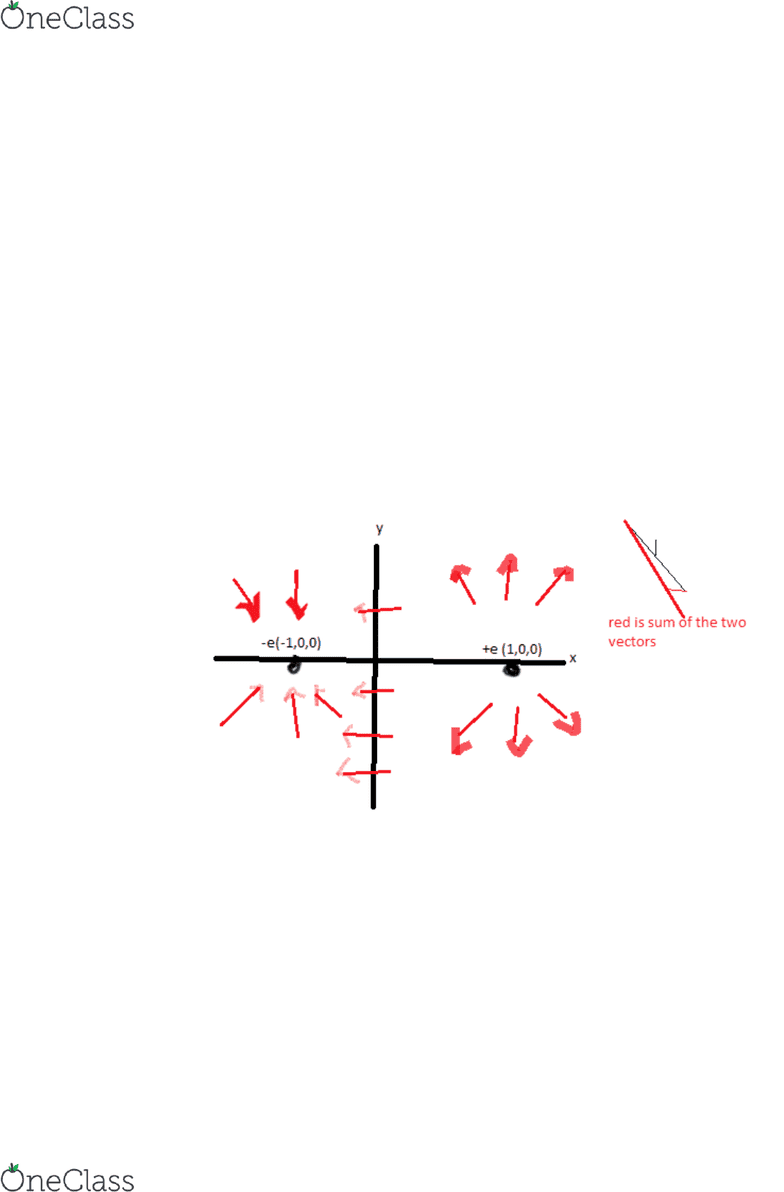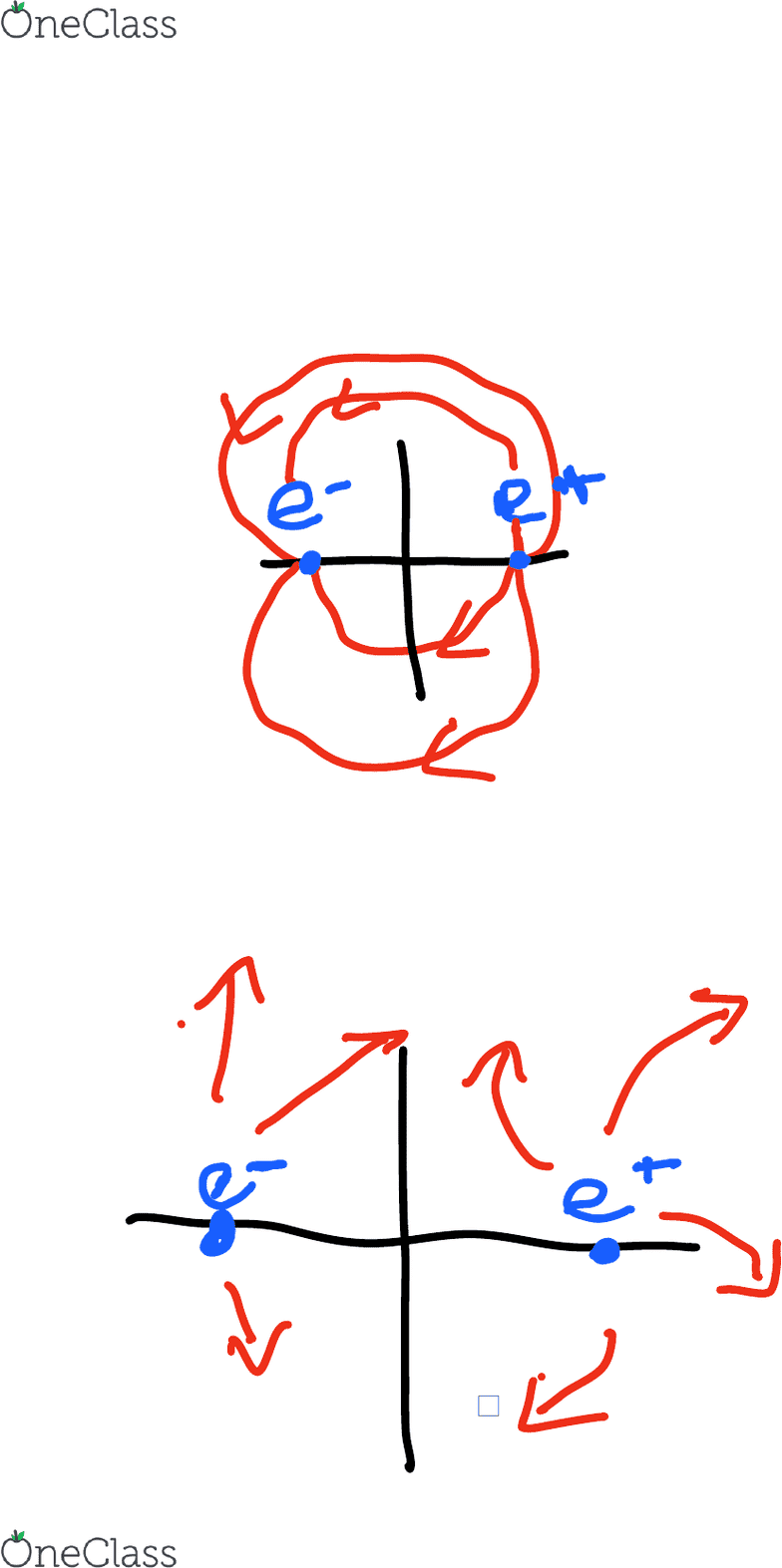# [PHYS 1010] - Midterm Exam Guide - Everything you need to know! (49 pages long)

772 views49 pages
School
York University
Department
Physics and Astronomy
Course
PHYS 1010
ProfessorYork
PHYS 1010
MIDTERM EXAM
STUDY GUIDE
Unlock document

This preview shows pages 1-3 of the document.
Unlock all 49 pages and 3 million more documents.Unlock document

This preview shows pages 1-3 of the document.
Unlock all 49 pages and 3 million more documents.Lecture 20B January 9th 2017
Slide 20B2
Electric field(E)= force(F)/ charge (q) or K(constant(charge object 1(q1))
(charge object 2(q2))/r^2, make the q into a vector by r^ (r hat)
K=(8.99 x10^9 N/m^2 )q=(1.602x10^-19Coulomb)/r^2 (r^ radially
outward from proton)
If sketching we would draw an arrow pointing away from the point in
middle. The closer we get to the proton, the smaller r is so the electric
field is bigger ( sorter is weaker and longer is stronger)
What would a proton do? Use this to create the diagram.
Slide 20.B.3
Calculations: E=(k(-e)/re-^2)r^) + (k(+e)/re+^2 r^ )( we need two terms
because we are checking for both)
We can look up K number in textbook
=-ke/1m^2 I^ (ihat) + ke/(1m)^2 -i^
=(-2ke/1m) I^
=-2(8.99 x 10^9 N/m^2/C^2)(1.602x10^-19C)/(1m^2)^2
=-2.88 x10^-9 N/c I^
Question (-1.11m,0.00,0.00)
=-ke/0.11m^2 -I^ (ihat) + ke/(2.11m)^2 -i^
= (1.19x10^-7 N/c i^)
Lecture 2
January 9, 2017
8:34 PM
Physics 1010 Lecture Page 1
find more resources at oneclass.com
find more resources at oneclass.com
Unlock document

This preview shows pages 1-3 of the document.
Unlock all 49 pages and 3 million more documents.= (1.19x10^-7 N/c i^)
The proton would move to the plus I^ direction ( toward -e) because it is
attracted to that and it would be longer since its really close to the field.
Question (0.00,1.00m,0.00)
= -ke/(sqroot(2m)^2(I^/2 +j^/2) + ke/(sqroot2m)^2 (-i^/sqroot2 +
j^/sqroot2)
=-k (8.99x 10^9) e(1.6 x 10^-19)/sqroot2 i^ N/c
=-1.02x10^-9N/C
Slide 20b.4
Physics 1010 Lecture Page 2
find more resources at oneclass.com
find more resources at oneclass.com
Unlock document

This preview shows pages 1-3 of the document.
Unlock all 49 pages and 3 million more documents.

## Document Summary

Electric field(e)= force(f)/ charge (q) or k(constant(charge object 1(q1)) (charge object 2(q2))/r^2, make the q into a vector by r^ (r hat) K=(8. 99 x10^9 n/m^2 )q=(1. 602x10^-19coulomb)/r^2 (r^ radially outward from proton) If sketching we would draw an arrow pointing away from the point in middle. The closer we get to the proton, the smaller r is so the electric field is bigger ( sorter is weaker and longer is stronger) Calculations: e=(k(-e)/re-^2)r^) + (k(+e)/re+^2 r^ )( we need two terms because we are checking for both) We can look up k number in textbook. The proton would move to the plus i^ direction ( toward -e) because it is attracted to that and it would be longer since its really close to the field. = -ke/(sqroot(2m)^2(i^/2 +j^/2) + ke/(sqroot2m)^2 (-i^/sqroot2 + j^/sqroot2) =-k (8. 99x 10^9) e(1. 6 x 10^-19)/sqroot2 i^ n/c.